407 493 6601

# Precalculus - The Unit Circles

## Introduction

• A Unit Circle is a type of circle whose center is at and whose radius length is equal to unit.
• This circle is modeled by the equation ${x}^{2}+{y}^{2}=1$. This equation is derived using the distance formula.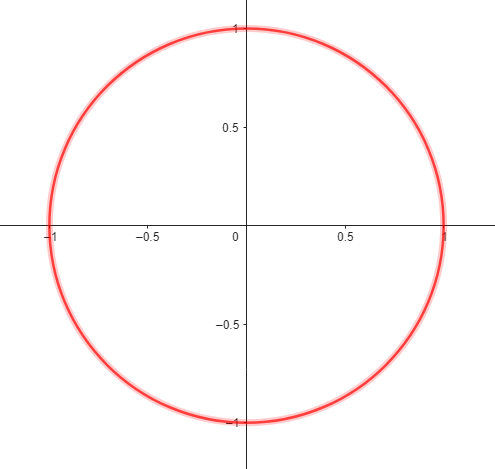• The circumference of the unit circle is equal to $2\mathrm{\pi }$ units or approximately equal to units.
• To inspect whether a point  is on the unit circle, we check the coordinates if they satisfy the equation ${x}^{2}+{y}^{2}=1$.
• The standard arc on the circle starts at the point  and travels counterclockwise if it is positive and clockwise if it is negative.
• Since the circumference of the unit circle is $2\mathrm{\pi }$, then the arc lengths are rational multiples of $2\mathrm{\pi }$
• Consider the unit circle being divided by the coordinate axes into four congruent arcs.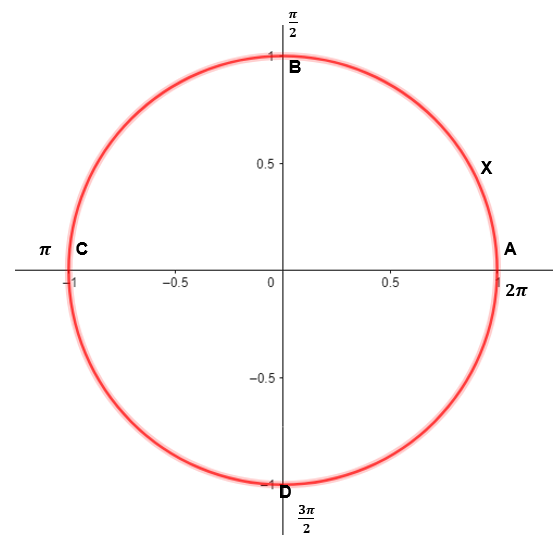• As point X moves from point A counterclockwise and terminates at point B, the length of arc $AB$ is $\frac{1}{4}$ of $2\mathrm{\pi }$ or $\frac{\mathrm{\pi }}{2}$.
• The length of arc $ABC$ is one-half of the circumference, which is $\mathrm{\pi }$.
• The length of arc $ACD$ is $\frac{3}{4}$ of the circumference, which is $\frac{3\mathrm{\pi }}{2}$.
• If we consider $\theta$ as the length of the arc, then we have the following:
•
• $X\left(\theta \right)=X\left(\mathrm{\pi }\right)=\left(-1,0\right)$
•

## Properties of the Unit Circle and the Special Angles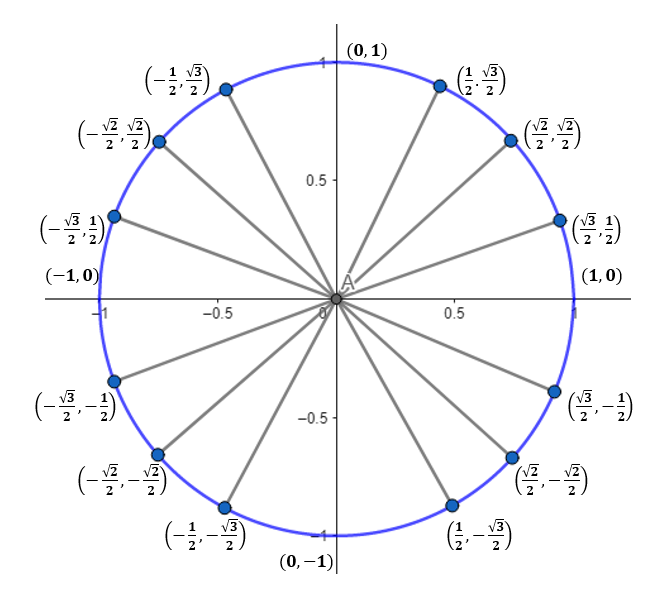• As shown in the figure, each point on the unit circle satisfies the equation ${x}^{2}+{y}^{2}=1$.
• Each point on the circle has coordinates corresponding to a special angle or multiple of special angles, such as and $90°$.
• Study the tables below.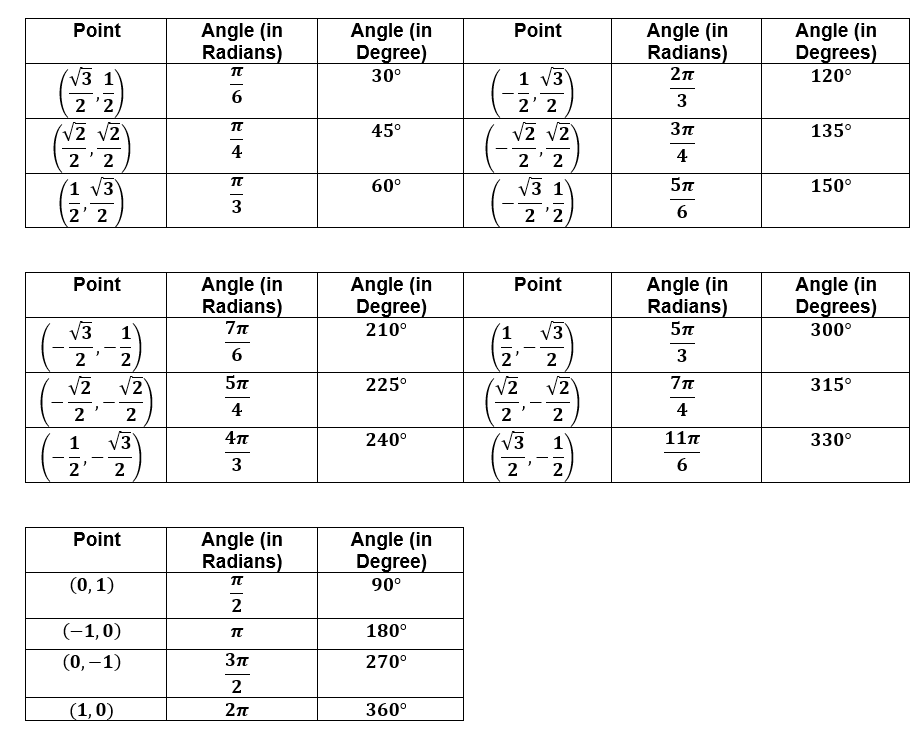• The domain of the unit circle ${x}^{2}+{y}^{2}=1$ is $\left\{x|x\in \mathrm{ℝ},-1\le x\le 1\right\}$. The range is $\left\{y|y\in \mathrm{ℝ},-1\le y\le 1\right\}$.
• Every real number (arc length) is associated uniquely with the central angle $\theta$ that subtends the standard arc on the unit circle.
• The relationship $P\left(\theta +2k\pi \right)=P\left(\theta \right)$, for any integer $k$ is called the Periodic Theorem. Typical points on the unit circle range from $0°$ to $360°$. Once the angle of the circular function exceeds $360°$, then this multiple of $360°$ has to be subtracted from the given angle. The difference is then equivalent to one common value between $0°$ to $360°$.

## Trigonometric Functions and the Unit Circle

• Let us consider a real number $\theta$ and let  be a point on the unit circle corresponding to the angle $\theta$.
• For each arc length on the unit circle, with the starting point  and terminal point , the values of and are real numbers, then we have $\mathrm{cos}\left(\theta \right)=x$ and $\mathrm{sin}\left(\theta \right)=y$.
• Study the figure below.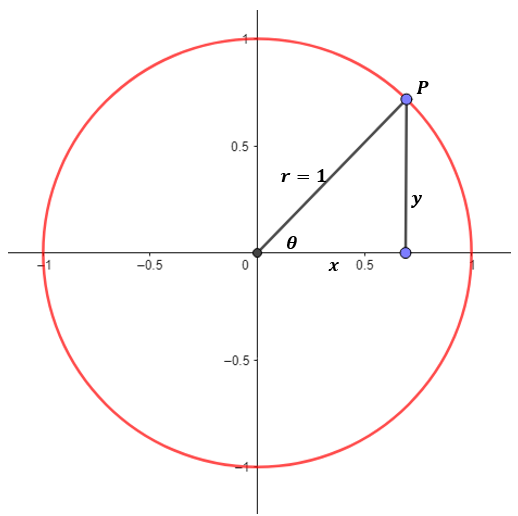• Point $P\left(x,y\right)$ is equal to $P\left(\mathrm{cos}\left(\theta \right),\mathrm{sin}\left(\theta \right)\right)$. This indicates that $\mathrm{sin}\left(\theta \right)=y$ and $\mathrm{cos}\left(\theta \right)=x$. This is based on $\mathrm{sin}\left(\theta \right)=\frac{y}{r}=\frac{y}{1}=y$ and $\mathrm{cos}\left(\theta \right)=\frac{x}{r}=\frac{x}{1}=x$
• Using the y and x coordinates, we can define the other trigonometric functions of $\theta$.
• , and .
• Trigonometric Functions are also called Circular Functions or Periodic Functions because they behave in a cyclic or repetitive manner.
• To illustrate, we have $\mathrm{sin}\left(\theta +2\pi \right)=\mathrm{sin}\left(\theta \right)$ and $\mathrm{cos}\left(\theta +2\pi \right)=\mathrm{cos}\left(\theta \right)$ as these functions have a period of $2\pi$.

Trigonometric Functions of Any Angle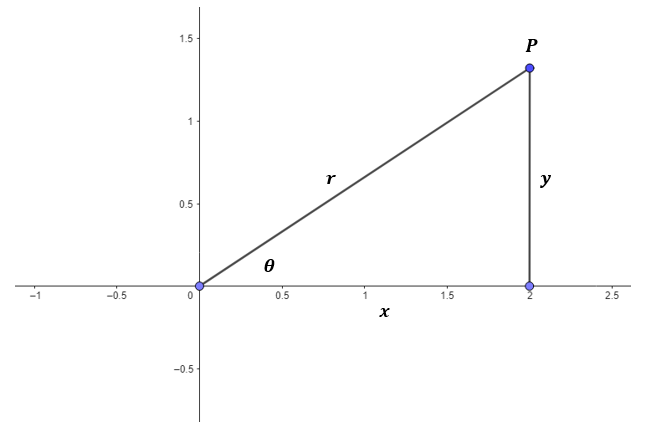• If we let $\theta$ be any angle in standard position, it indicates that its vertex is at the origin and the initial side is the positive x-axis.
• The six trigonometric functions are described in the tables below, and their respective signs in the four quadrants.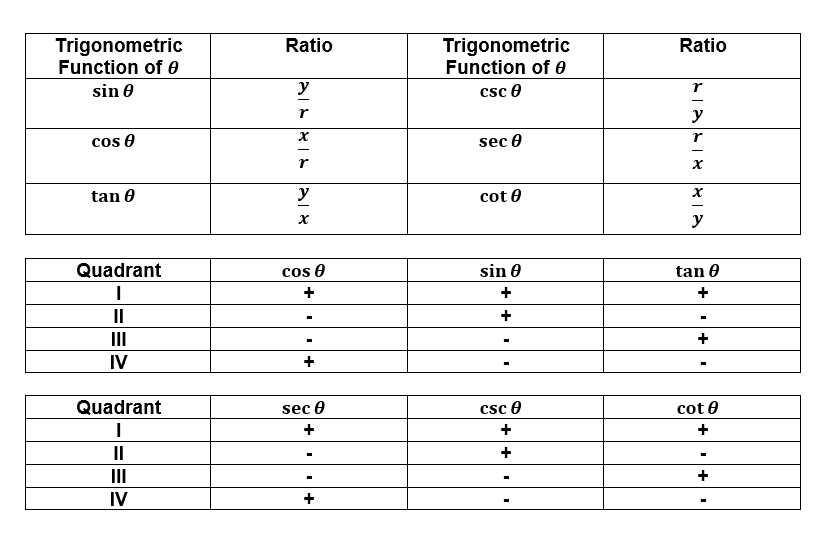Reference Angle

• For any angle $\theta$, its reference angle is the acute angle that the terminal side of $\theta$ makes with the positive x-axis or negative x-axis.
• If $0°<\theta <90°$, then the reference angle $\beta$ is equal to $\theta$.
• In Quadrant II, the reference angle is $\beta =180°-\theta$.
• In Quadrant III, the reference angle is $\beta =\theta -180°$.
• In Quadrant IV, the reference angle is $\beta =360°-\theta$.

In general, the angle $\theta$ and where $n\in \mathrm{ℤ}$ have the same terminal side. These angles are called co-terminal angles.

## Solved Examples

Example 1. Find the exact value of $\mathrm{cos}\left(\frac{13\mathrm{\pi }}{6}\right)$ using the standard unit circle.

Solution:

$\frac{13\mathrm{\pi }}{6}=390°$

$\frac{13\mathrm{\pi }}{6}$ terminates in Quadrant I, which forms $30°$ in the positive x-axis.

Consider the angle and choose the point $\left(\frac{\sqrt{3}}{2},\frac{1}{2}\right)$, on the unit circle.

This shows that $\mathrm{cos}\left(\frac{13\mathrm{\pi }}{6}\right)=\mathrm{cos}\left(\frac{\mathrm{\pi }}{6}\right)=\frac{\sqrt{3}}{2}$

Note that the sign is positive since $\mathrm{cos}\left(\theta \right)>0$ in Quadrant I.

Example 2. Given that $\mathrm{tan}\left(\theta \right)=\frac{5}{12}$ and $\mathrm{sin}\left(\theta \right)<0$, find the other trigonometric functions of $\theta$.

Solution:

If the tangent function is positive and sine function is negative, then $\theta$ is Quadrant III.

$\mathrm{tan}\left(\theta \right)=\frac{y}{x}=\frac{5}{12}$

${r}^{2}=\sqrt{{x}^{2}+{y}^{2}}\to {r}^{2}=\sqrt{{\left(12\right)}^{2}+{\left(5\right)}^{2}}$

$r=13$

## Cheat Sheet

• Trigonometric functions of different special angles are summarized in the tables below.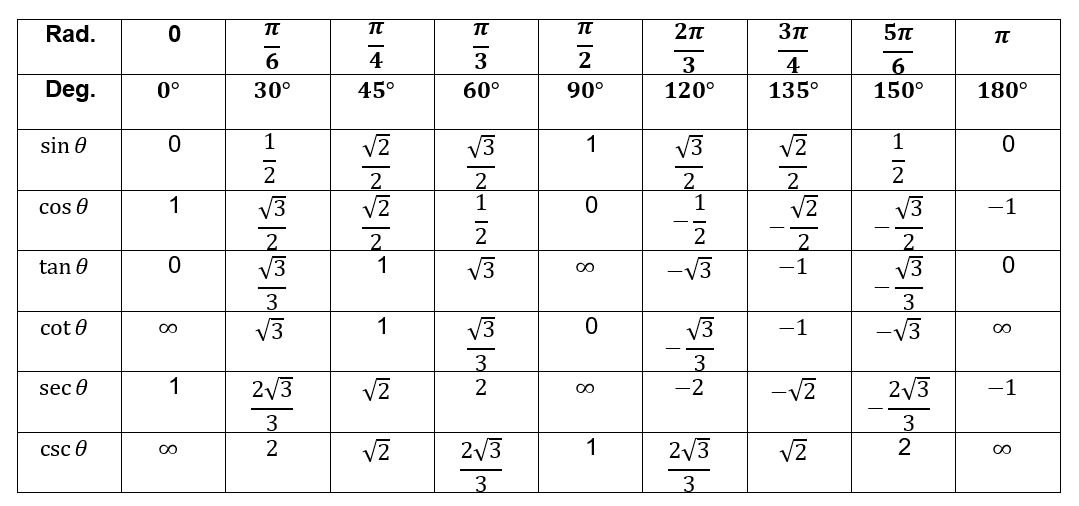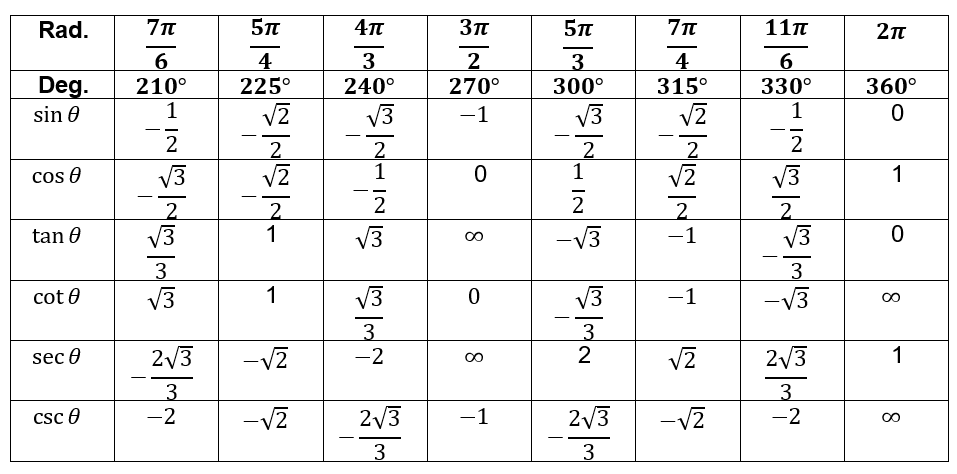• The cosine function and secant functions are even. To illustrate, we have $\mathrm{cos}\left(-\theta \right)=\mathrm{cos}\left(\theta \right)$ and $sec\left(-\theta \right)=sec\left(\theta \right)$.
• The sine, cosecant, tangent, and cotangent functions are odd.

## Blunder Areas

• Always be mindful of the sign of each trigonometric function in the four quadrants.
• Reference angles can be used to evaluate trigonometric functions.
• Suppose that $\mathrm{tan}\left(\theta \right)=-\frac{1}{3}$ and we want to find the other trigonometric values of $\theta$, we solve for the radius. The radius is always positive.
• The angles associated with trigonometry (in the unit circle approach) are directed angles. Angles obtained from the counterclockwise direction are positive while angles obtained from the clockwise direction are negative.